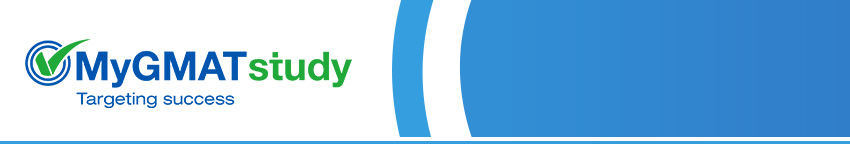# Quantitative SectionEnroll Now Name:* Email (User ID):* Phone Number:* Institute Graduated From:* Graduation Year:*

Arithmetic  Properties of Numbers, Odds and Evens, Primes and Divisibility, Powers and Roots, Inequalities, and Fractions and Decimals.

Algebra  Fundamentals of Algebra, Linear and Simultaneous Equations, Quadratic Equations, and Set Theory.

Combinatorics & Probability - Descriptive Statistics, Permutation and Combination, and Probability.

Geometry  Plane Geometry, Coordinate Geometry, Solid Geometry.

Word Problems  Averages, Percentages, Profit and Loss, Simple and Compound Interest, Ratios and Mixtures, Proportions and Variations, Time, Speed and Distance, Time and Work.

Over a period of years, we have observed that the GMAT places special emphasis on application-based questions rather than pure mathematical concept-based problems. At MyGMATstudy, we have identified periodic pattern shifts in the GMAT and prepared the chapter guides and tests accordingly.

The package is attractively priced at \$150 only.